# No more fear of Pointers

## Variable Allusion | Pointers’ Primer

The concept of pointers is fundamental to programming. These are the ones that are most feared and misunderstood. The phrase “A little knowledge is a dangerous thing” perfectly suits pointers.

Pointers are challenging to grasp at first. Everything you started from the beginning is difficult as well!

Extended pointer features are supported in C and C++. Other languages adopted a subset of pointer features or variants.

No matter which language you are into, it is still worth understanding.

This article assumes that you are familiar with basic C or C++ programming. It’s quite a lengthy read.

## 🚉 First Stop — Variable

A variable is a memory container where value resides.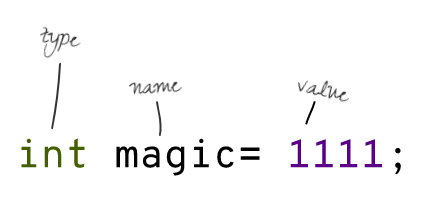A variable can be associated with:

• A name is what you call the variable; it is an essential part of communication among programmers.
• A type defines the domain of values that can be stored inside the variable, encoding scheme (for primitive type), and the container’s memory size.
• An address is the location of a variable in memory; it may be physical or logical.

Each byte in memory has an address that the processor may use to read or write data.

A variable may span multiple bytes. A variable of `int` type holds `4 bytes` in memory (`4 bytes` span); it has four addresses, but we always use its first-byte address.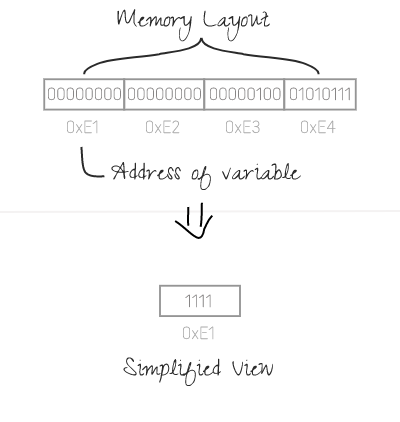The address of a variable always means the address of its first byte.

### Why do we address a variable by its first-byte address? 🤔

To keep things simple. Moreover, determining all of the related addresses is easy... If the size and the starting address of a variable are known.

The size of a variable is defined by its type. According to established conventions, a multi-byte primitive typed variable is always stored in contagious places.

So, if the address of a `four-byte int` variable is `10`, the other three addresses are `11`, `12`, and `13`.

## 🚉 Last Stop — Pointer

A Pointer is a variable that points to another variable. Technically, A Pointer’s value is another variable’s memory address (the starting address).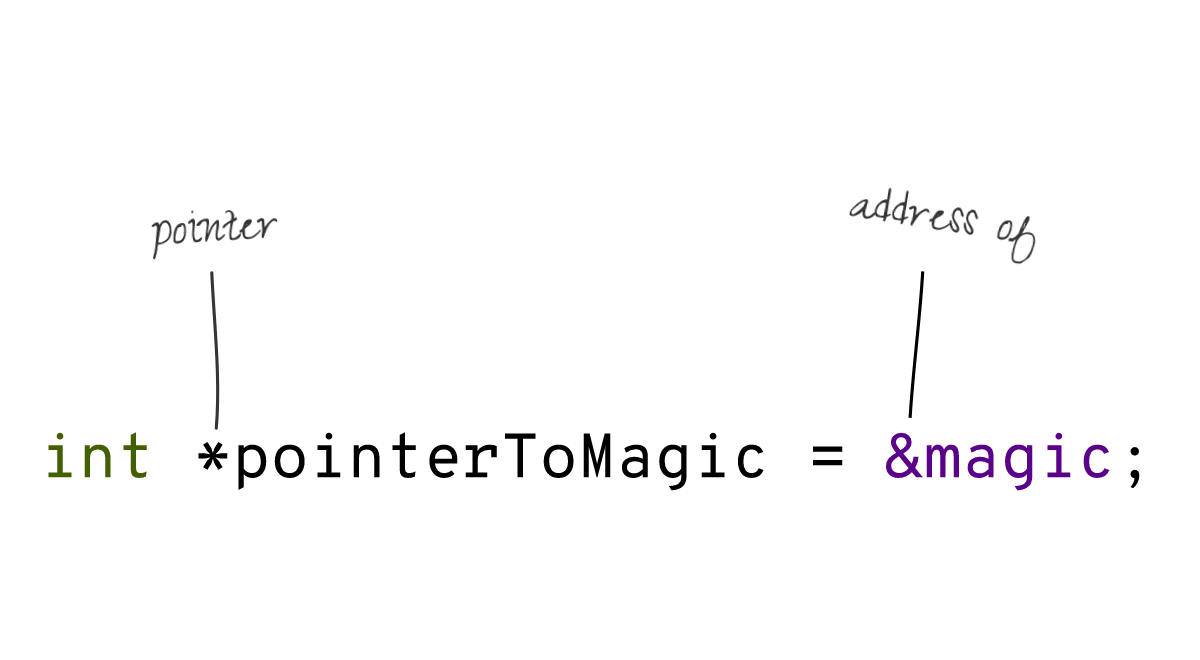Pointers are easy to follow if these are visualized graphically.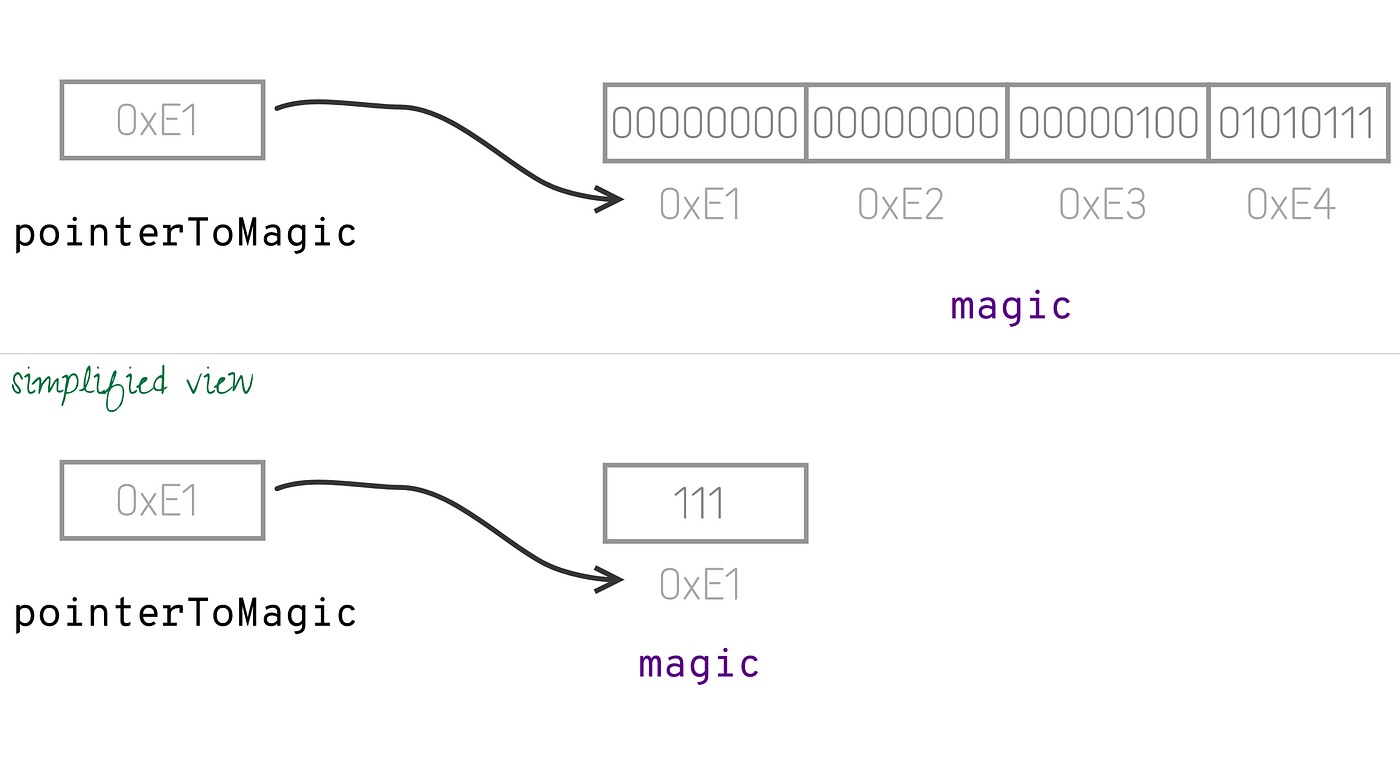### Pointers Declaration

``````// --- Example-1 ---
int a = 2;
int *pointerToA = &a; // declaration and defination of pointer;

// --- Example-2 ---
int *pointerToB; //declaration
int b = 3;

// * is only used for declaration.
pointerToB = &b; //defination

// --- Example-3 ---
int *pointerToArray;
int array[] = {1, 2, 3};

// & is not used in case of array.
// Remember array name itself a pointer to first element
pointerToArray = array;

// --- Example-4 ---
char *pointerToString;

// & is not used again. Remember a string is an array.
pointerToString = "hello";

// --- Example-5 ---
// declaration of two pointers
int *p1, *p2; // repeat * to declare another pointer.

// --- Example-6 ---
int* p1, v; // only p1 is pointer, v is interger variable
``````

A memory address is a number, so the type of a pointer is always Integer despite its pointing.

If a pointer is an integer, then what about the type we set at the time of the declaration?
It’s the referenced variable’s type, and it’s only necessary if pointer arithmetic is required; otherwise, it’s not. Call it: “declared pointer type” for the sake of brevity. `void *` (Generic pointer) is used if the referred variable type is not known.

### Why we need pointers?

A pointer facilitates indirect access to a variable. This feature enables the passing of variables to functions efficiently.

Typically, when we pass values to a function, these values are copied to function parameters. It can be understood as:

``````int first = a; // value of a is copied to first
int second = 2; // 2 is copied to second
``````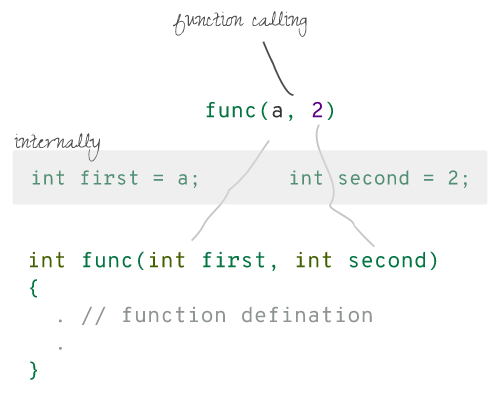Copying isn’t a problem for scalar (single-valued) kinds, but it would be a performance hit for multi-valued types (string, array, custom type).

So what is the solution? Send the values indirectly. Pointers facilitate the indirect passing of the values.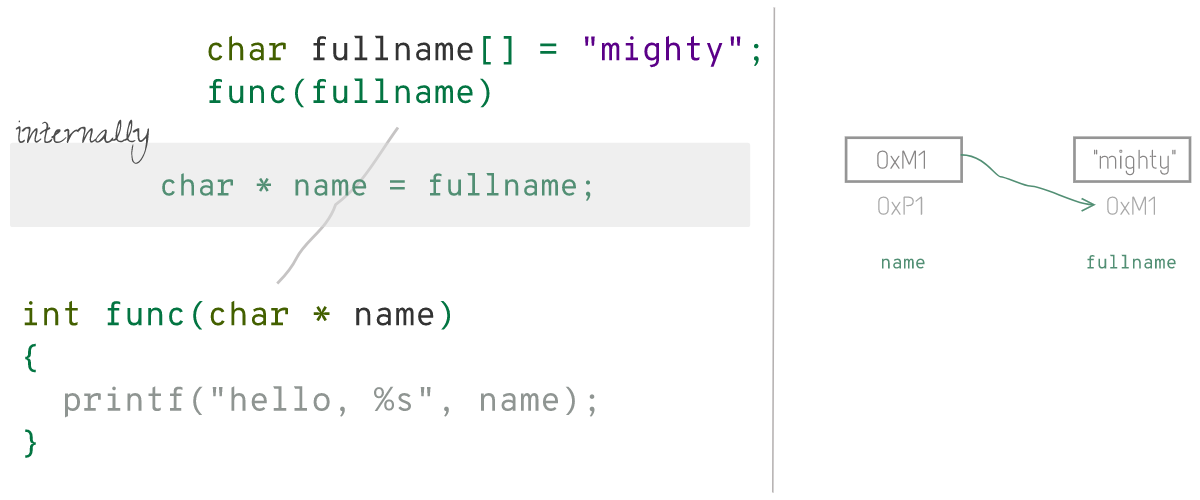### How do I access the referred variable's value back through the pointer?

Value-at or Deference operator is used to access the variable to which a pointer is pointing. Sadly, symbol `*` (asterisk) has been reused for this purpose.

Let's see it in action:

``````int a = 5;
int *pointerToA = &a;

a = 6;

// * is used as value-at operator
// whenever you use * outside declaration syntax, it is value-at symbol

// accessing value through pointer
printf("%d\n", *pointerToA); // print 6

// writing value to pointer
*pointerToA = 8;
printf("%d\n", a); //print 8

// taking new value from user
scanf("%d", pointerToA); // equivalent to scanf("%d", &a);

char hello[] = "hello";
char * pointerToHello =  hello;

// missing of '*' here : not needed for array or string
printf("%s\n", pointerToHello);
``````

In the case of string and array, the value can be access through `[]` index-of operator. The following expression is equivalent:

``````pointer[i] = *(pointer + i)  (see next section)
``````

You can interchange an array with a pointer for accessing and updating values.

An Array name is a pointer to its first element.

### Thinking Mathematically

The beauty of pointers is that you can visualize their assignment statement as algebraic equations.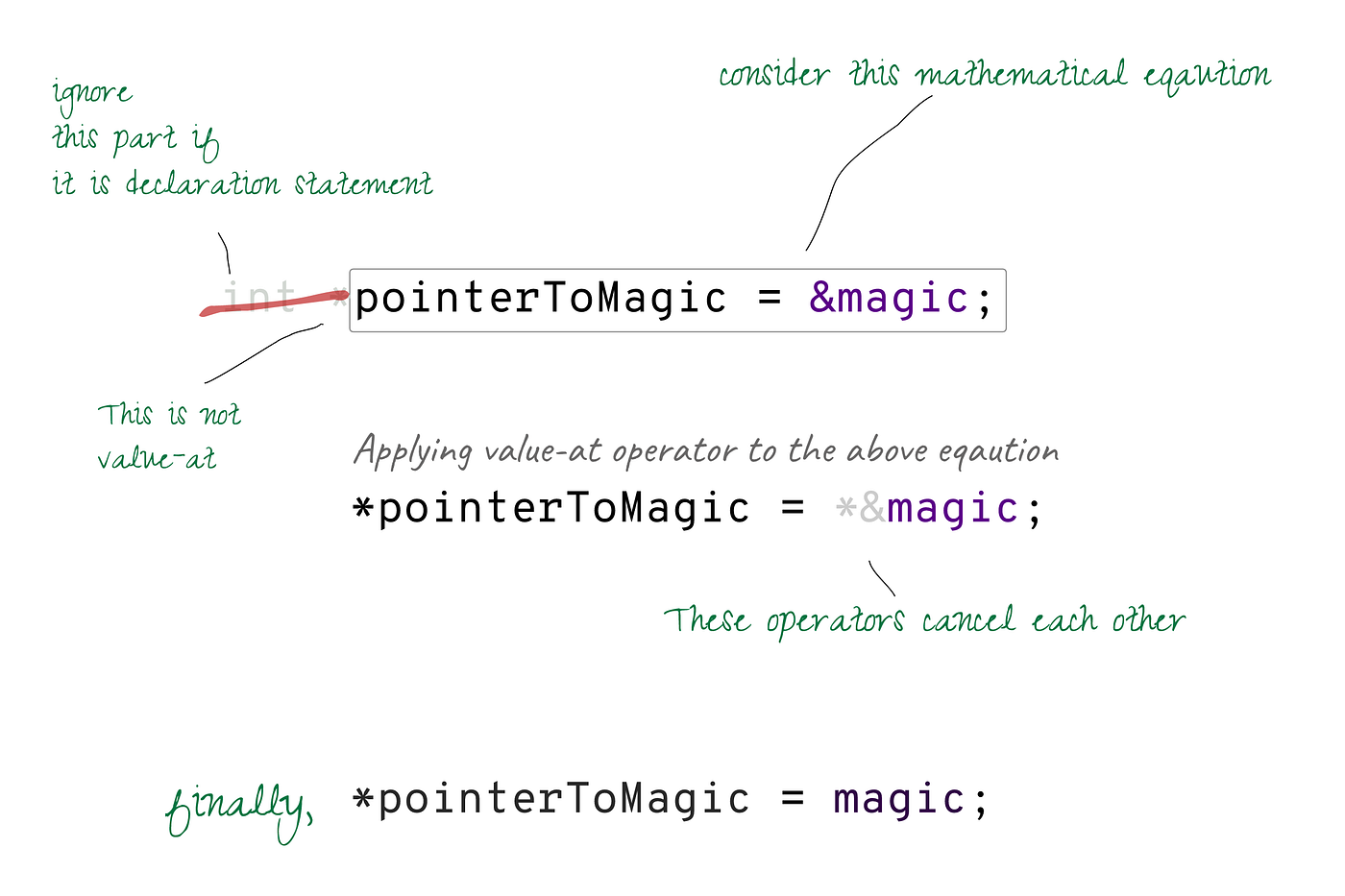By thinking this way, you can get cozy with pointers to any level 😉.

``````int a = 5;
int *pointerToA = &a;

int **pointerToPointerToA = &pointerToA;

printf("%d", a); // print 5

// pointerToA = &a; __eq0
// *pointerToA = *&a; // applying * (value-at)
// *pointerToA = a; // * and & cancel each other
// *pointerToA = a; __eq1
print("%d", *pointerToA); // print a's value

// pointerToPointerToA = &pointerToA; __eq2
// *pointerToPointerToA = pointerToA; // applying *
// **pointerToPointerToA = *pointerToA; // applying ** again
// thus **pointerToPointerToA = a __from eq1
print("%d", **pointerToPointerToA); //print a's value
``````

### Pointers Arithmetic

An integer can be added or subtracted to/from a pointer. You may use this construct to iterate across an array or a string. But keep in mind that you can only conduct this calculation if you've specified the pointer's declared type. Adding a number to a pointer follows the following equation:

``````new-location = old-location + number x sizeof(<type you declared>);
``````

Visually, it can be imagined as: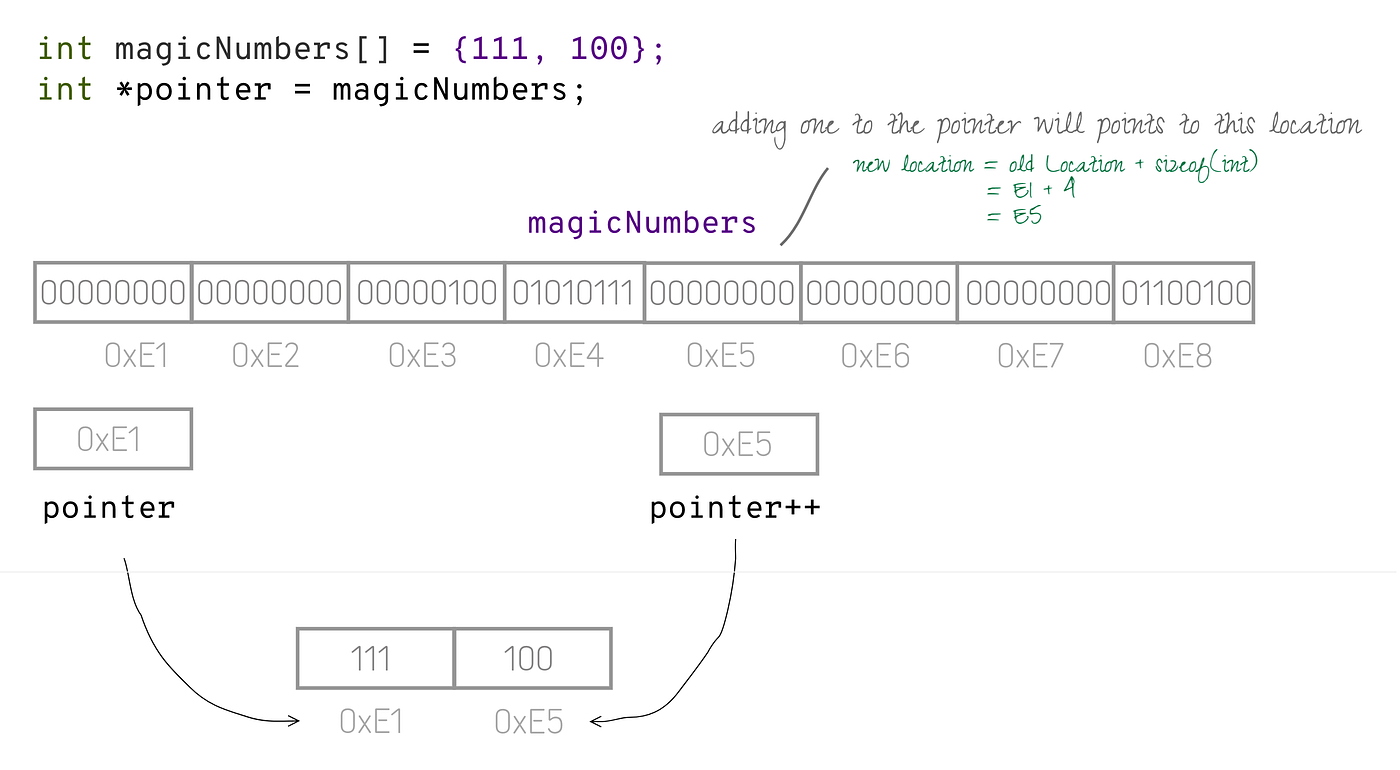``````// Calculating the length of string
char *hello = "hello";
char *i = hello; // i point to hellow first character
int length = 0;
while (*i != '\0') {

length++;
i++; // pointer addtion: move to next character
}

printf("length of %s is %d", hello, length);
``````

### Dynamic allocation of memory

Pointers also enable us to create dynamically sized memory buffers or arrays or string.

``````int size = 10;
// malloc from stdlib.h : allocates chunks of memory
char * dynamicSizedString= malloc(size * sizeof(char));
scanf("%s", dynamicSizedString);

int *dynamicSizedArray = malloc(size * sizeof(int));

for (int i = 0; i < size; i++) {
scanf("%d", &dynamicSizedArray[i]);
// scanf("%d", dynamicSizedArray+i); //
}
``````

### 😎 Show off your skills with Pointers to Function

Pointers can also point to Functions, which helps in passing Functions to Functions.

``````type (*function_pointer)(parameters)
``````
``````void sort(int * numbers, int (*compare)(int, int)) {

...

int r = compare(a, b) // calling compare | no '*' symbol

}

int asc(int a, int b) {
return a - b;
}

int desc(int a, int b) {
return b - a;
}

// Note function name is pointer just like array | no '&' symbol in assignment
// sort by asc | *compare = asc | no '&' symbol
sort(numbers, asc)

// sort by desc | *compare = desc
sort(numbers, desc)

``````

A pointer can also point to user-defined types: `class`, `struct`

``````// class : C++
Employee *pointerToEmployee = new Employee();
// calling member method
double salary = (*pointerToEmployee).getSalary();
``````

A member method or field can also be accessed using Arrow Operator `->`. It can be thought as transformation `(*pointer).member` to `pointer->member`.

``````// class : C++
Employee *pointerToEmployee = new Employee();
// calling member method
double salary = pointerToEmployee -> getSalary();
``````

Some definitions are associated with pointers:

• Dangling Pointer points to a location that is no more valid and accessing such location cause segmentation fault.
• Null Pointer does not point to any memory location/variable.

Wherever possible, visualize pointers graphically and deals with them mathematically to avoid unnecessary confusion and headaches.

## Pointers Beyond C and C++

It is wrong to say that languages other than C and C++ do not support pointers. Most languages do support, but not extensively as C and C++ do.

• In languages like Java, JavaScript, References are pointers equivalent. References can be considered the mini version of pointers with reduced features: simplified syntax, no arithmetic.
• In language GO, pointers have similar syntax and allowed operations (except pointer arithmetic).

## Takeaways

• Pointers provide a mechanism to indirectly access variables or functions.
• An array name is a pointer to its first element.
• `*` is used to declare pointer if used with type, and the same symbol is also used to de-reference the pointer.
• Pointers can be added or subtracted, provided declared type is defined.
• Pointers allow creating dynamic arrays.
• `->` operator is used to access type member: field or method.
• A Null Pointer does not point to anything, and a Dangling pointer stores an invalid address.